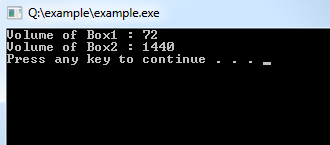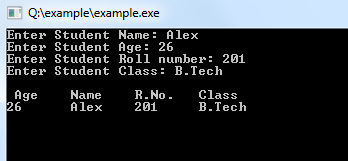## C++ Programming Tutorial Index

In object-oriented programming languages like C++, the data and functions (procedures to manipulate the data) are packed together as a self-contained unit called a class. This tutorial explains how to use C++ classes.

Class is an extended concept similar to the `struct` in the C programming language. In C++, a class describes objects' properties (data) and behaviors (functions). Classes are not objects, but they are used to instantiate objects.

## What is a Class in C++?

• Class is an abstract data type similar to the C structure.
• A class is a representation of objects and some set of operations that can apply to such objects.
• The class consists of Data members and methods.
• The primary purpose of a class is to hold data/information. This is achieved with attributes which are also known as data members.
• The member functions determine the class's behavior, i.e., provide a definition for supporting various operations on data held in the form of an object.

### Class Definition

Syntax:

``````Class class_name
{
Data Members;
Methods;
}``````

Example:

``````class A
{
public:
double length; // Length of a box
double height; // Height of a box
}``````
• `private`, `protected`, and `public` is called visibility labels.
• The members that are declared private can be accessed only from within the class.
• Public members can be accessed from outside the class also.
• In C++, data can be hidden by making it private.

## Class Members

Data and functions are members. Data Members and methods must be declared within the class definition.

Example:

``````Class A
{
int i; // i is a data member of class A
int j; // j is a data member of class A
int i; // Error redefinition of i
}``````
• A member cannot be redeclared within a class.
• No member can be added elsewhere other than in the class definition.

Example:

``````Class A
{
int i;
int j;
void f (int, int);
int g();
}``````

`f` and `g` are a member function of `class A`. They determine the behavior of the objects of `class A`.

### Accessing Data Members

The `public` data members of objects of a class can be accessed using the direct member access operator (`.`). Let us try the following example to make things clear:

Example:

``````#include <iostream>
using namespace std;

class Box
{
public:
double length; // Length of a box
double height; // Height of a box
};

int main( )
{
Box Box1; // Declare Box1 of type Box
Box Box2; // Declare Box2 of type Box
double volume = 0.0; // Store the volume of a box here

// box 1 specification
Box1.height = 4.0;
Box1.length = 6.0;

// box 2 specification
Box2.height = 10.0;
Box2.length = 12.0;

// volume of box 1
volume = Box1.height * Box1.length * Box1.breadth;
cout << "Volume of Box1 : " << volume <<endl;

// volume of box 2
volume = Box2.height * Box2.length * Box2.breadth;
cout << "Volume of Box2 : " << volume <<endl;
return 0;
}``````

Program Output:It is important to note that `private` and `protected` members cannot be accessed directly using a direct member access operator (`.`). We will learn how `private` and `protected` members can be accessed.

#### Program to Enter Student’s Details and Display It

Example:

``````#include <iostream>
using namespace std;

class stud
{
public:
char name,clas;
int rol,age;

void enter()
{
cout<<"Enter Student Name: "; cin>>name;
cout<<"Enter Student Age: "; cin>>age;
cout<<"Enter Student Roll number: "; cin>>rol;
cout<<"Enter Student Class: "; cin>>clas;
}

void display()
{
cout<<"\n Age\tName\tR.No.\tClass";
cout<<"\n"<<age<<"\t"<<name<<"\t"<<rol<<"\t"<<clas;
}
};

int main()
{
class stud s;
s.enter();
s.display();
cin.get();  //use this to wait for a keypress
}
``````

Program Output: